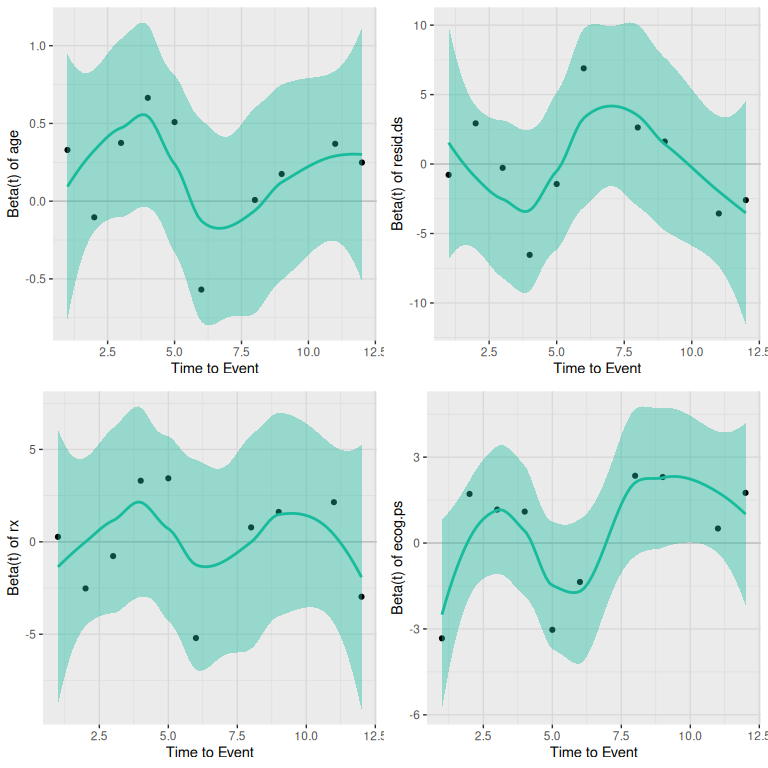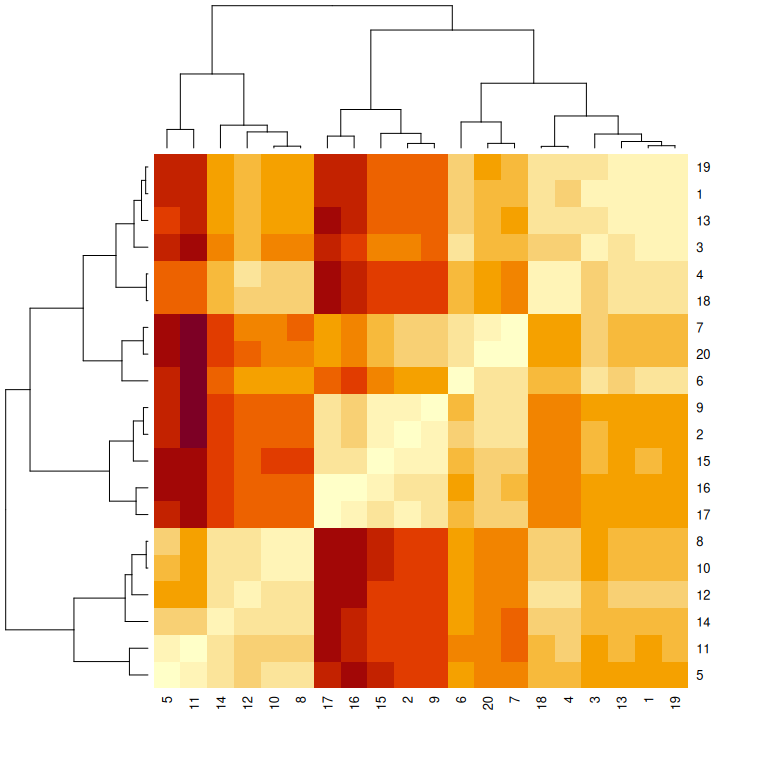knitr::opts_chunk$set(echo = TRUE) library(CaseBasedReasoning) ## Lade nötiges Paket: Rcpp ## Lade nötiges Paket: RcppParallel ## ## Attache Paket: 'RcppParallel' ## Das folgende Objekt ist maskiert 'package:Rcpp': ## ## LdFlags ## Lade nötiges Paket: rms ## Lade nötiges Paket: Hmisc ## ## Attache Paket: 'Hmisc' ## Die folgenden Objekte sind maskiert von 'package:base': ## ## format.pval, units ## Lade nötiges Paket: survival ## Lade nötiges Paket: lattice ## Lade nötiges Paket: ggplot2 ## Lade nötiges Paket: SparseM ## ## Attache Paket: 'SparseM' ## Das folgende Objekt ist maskiert 'package:base': ## ## backsolve library(survival) # 1 Motivation This section demonstrates how to apply Cox Proportional Hazards (CPH) regression for Case-Based Reasoning (CBR). CPH regression is a popular survival analysis technique that models the relationship between the hazard rate and the predictor variables while accounting for the time-to-event data. Linear and logistic regression models can be employed similarly for CBR by initializing the models as follows: 1. Data Preparation: Begin by preparing the dataset, ensuring that it includes the relevant features and the time-to-event data. For CPH regression, you’ll need to include a column indicating the event status (e.g., 1 for the event occurring and 0 for censored observations). For linear and logistic regression models, prepare the dependent and independent variables accordingly. 2. Feature Selection: Identify the most relevant features or predictor variables for the regression models. Techniques like correlation analysis, mutual information, or machine learning algorithms can be used to select the most important features for the CBR process. 3. Model Initialization: Initialize the appropriate regression model for the analysis: cph_model <- CoxModel$new(Surv(futime, fustat) ~ age + resid.ds + rx + ecog.ps, ovarian)

# linear_model <- LinearBetaModel$new(y ~ x1 + x2 + x3) # logistic_model <- LogisticBetaModel$new(y ~ x1 + x2 + x3)
1. Model Training: Fit the initialized regression model to the training data.
cph_model$fit() 1. Model Evaluation: Evaluate the performance of the fitted regression models using appropriate evaluation metrics, such as concordance index for CPH regression, R-squared for linear regression, or accuracy and AUC-ROC for logistic regression. 2. Similarity Assessment: Use the trained regression models to assess the similarity between cases by comparing the predicted outcomes or coefficients of the predictor variables. Techniques such as distance metrics or clustering algorithms can be employed to group similar cases. 3. Retrieve, Reuse, Revise, and Retain: After identifying the most similar cases using the trained regression models, follow the traditional CBR process. Adapt the retrieved cases to fit the new problem, evaluate the revised solution, and add the new case and solution to the database for future reference. By integrating regression models like CPH, linear, and logistic regression into the CBR process, it becomes possible to capture the relationships between variables more effectively, leading to more accurate identification of similar cases and improved problem-solving capabilities. # 2 Cox Proportional Hazard Model In the first example, we demonstrate the application of the Cox Proportional Hazards (CPH) model using the ovarian dataset from the survival package. To begin, we initialize the R6 data object, which is essential for managing and organizing the dataset. This step sets the foundation for further analysis, enabling efficient implementation of the CPH model in the Case-Based Reasoning process. ovarian$resid.ds <- factor(ovarian$resid.ds) ovarian$rx <- factor(ovarian$rx) ovarian$ecog.ps <- factor(ovarian$ecog.ps) # initialize R6 object cph_model <- CoxModel$new(Surv(futime, fustat) ~ age + resid.ds + rx + ecog.ps, ovarian)

In the preprocessing stage, all cases with missing values in the learning and endpoint variables are removed using the na.omit function, and the cleaned dataset is stored internally. A text output is provided, indicating the number of cases that were dropped due to missing values. Additionally, any character variables present in the data are converted to factor variables to ensure compatibility with subsequent statistical analyses.

# 3 Available Models

In the context of Case-Based Reasoning (CBR), linear regression, logistic regression, and Cox proportional hazards regression can be used for estimating the similarity between cases. Each of these regression methods has its own advantages and disadvantages, which are outlined below.

## 3.1Linear Regression

1. Simplicity: Linear regression models are easy to understand and interpret, which makes it straightforward to explain the model’s behavior to stakeholders.

2. Speed: Linear regression models are computationally efficient, requiring less processing power and time to fit compared to more complex models.

1. Limited to continuous dependent variables: Linear regression models can only be applied to problems with continuous dependent variables, which limits their applicability in CBR scenarios.

## 3.2Logistic Regression

1. Binary classification: Logistic regression is suitable for binary classification problems, making it more applicable in CBR scenarios where the outcome is binary (e.g., success or failure).

2. Interpretability: Logistic regression models provide insights into the relationship between variables through coefficients, allowing for easier interpretation of feature importance.

1. Limited to linear relationships: Logistic regression models assume a linear relationship between the independent variables and the logit transformation of the dependent variable, which may not always hold true in real-world situations.

## 3.3Cox Proportional Hazards Regression

1. Time-to-event data: Cox proportional hazards regression is specifically designed for time-to-event data, making it highly applicable in CBR scenarios where the outcome is a survival or failure event occurring over time.

2. Deals with censoring: Cox proportional hazards regression can handle right-censored data, which is common in survival analysis scenarios.

1. Proportional hazards assumption: Cox proportional hazards regression assumes that the hazard ratios are constant over time, which may not always hold true in real-world situations.

2. More complex: Cox proportional hazards regression is more complex compared to linear and logistic regression models, which can make explaining the model’s behavior to stakeholders more challenging.

In conclusion, the choice between linear regression, logistic regression, and Cox proportional hazards regression for CBR depends on the specific problem and dataset at hand. Factors such as the type of dependent variable (continuous, binary, or time-to-event), the presence of censoring, and the need for interpretability should all be considered when selecting the most appropriate method.

## 3.4Random Forests

1. Non-linear relationships: Random forests can capture complex, non-linear relationships between variables, making them suitable for a wider range of problems.

2. Robustness to outliers: Random forests are less susceptible to outliers compared to linear regression models, leading to more stable predictions.

3. Feature interactions: Random forests can capture interactions between features, which can be particularly useful in CBR when there are complex relationships between variables.

4. Reduced overfitting: Random forests employ an ensemble of decision trees, which generally results in reduced overfitting compared to single models, such as linear regression.

1. Complexity: Random forests are more complex and harder to interpret compared to linear regression models, which can make explaining the model’s behavior to stakeholders more challenging.

2. Computationally expensive: Random forests require more processing power and time to fit compared to linear regression models, particularly with large datasets and a high number of trees.

3. Less interpretable feature importance: Although random forests can provide feature importance scores, interpreting these scores can be more challenging compared to the coefficients of a linear regression model.

In conclusion, the choice between linear regression models and random forests for CBR depends on the specific problem and dataset at hand. Factors such as the complexity of relationships between variables, the presence of outliers, computational resources, and the need for interpretability should all be considered when selecting the most appropriate method.

# 4 Case Based Reasoning

## 4.1 Search for Similar Cases

Once the initialization is complete, the goal is to identify the most similar case from the training data for each case in the query dataset. This process involves comparing the features of the query cases with those of the training cases, using similarity measures or statistical models, to retrieve the most closely matched cases, thereby facilitating the Case-Based Reasoning process.

n <- nrow(ovarian)
trainID <- sample(1:n, floor(0.8 * n), F)
testID <- (1:n)[-trainID]

cph_model <- CoxModel$new(Surv(futime, fustat) ~ age + resid.ds + rx + ecog.ps, ovarian[trainID, ]) # fit model cph_model$fit()

# get similar cases
matched_data_tbl = cph_model$get_similar_cases(query = ovarian[testID, ], k = 3) knitr::kable(head(matched_data_tbl)) futime fustat age resid.ds rx ecog.ps scDist caseId 22 268 1 74.5041 2 1 2 0.3198076 1 1 59 1 72.3315 2 1 1 0.4332524 2 3 156 1 66.4658 2 1 2 1.9264911 3 19 1129 0 53.9068 1 2 1 0.2204346 1 5 431 1 50.3397 2 1 1 0.6154656 2 12 744 0 50.1096 1 2 1 0.7640594 3 After identifying the similar cases, you can extract them along with the verum data and compile them together. However, keep in mind the following notes: Note 1: During the initialization step, we removed all cases with missing values in the data and endPoint variables. Therefore, it is crucial to perform a missing value analysis before proceeding. Note 2: The data.frame returned from cph_model$get_similar_cases includes four additional columns:

1. caseId: This column allows you to map the similar cases to cases in the data. For example, if you had chosen k=3, the first three elements in the caseId column will be 1 (followed by three 2’s, and so on). These three cases are the three most similar cases to case 0 in the verum data.
2. scDist: The calculated distance between the cases.
3. scCaseId: Grouping number of the query case with its matched data.
4. group: Grouping indicator for matched or query data.

These additional columns aid in organizing and interpreting the results, ensuring a clear understanding of the most similar cases and their corresponding query cases.

## 4.2 Check Proportional Hazard Assumption

Lastly, it is important to check the Cox proportional hazard assumption to ensure the validity of the model. This assumption states that the hazard ratios between different groups remain constant over time. By verifying this assumption, you can confirm that the relationships captured by the model are accurate and reliable for the given dataset, ultimately enhancing the effectiveness of the Case-Based Reasoning process.

cph_model$check_ph()# 5 Distance Matrix Calculation Alternatively, you might be interested in examining the distance matrix to better understand the relationships between cases: distance_matrix = cph_model$calc_distance_matrix()
heatmap(distance_matrix)cph_model$calc_distance_matrix() computes the distance matrix between the train and test data. If test data is omitted, the function calculates the distances between observations in the train data. In the resulting matrix, rows represent observations in the train data, and columns represent observations in the test data. The distance matrix is stored internally in the CoxModel object as cph_model$distMat.

Visualizing the distance matrix as a heatmap can provide insights into the relationships between cases, assisting in the identification of similar cases and improving the Case-Based Reasoning process.